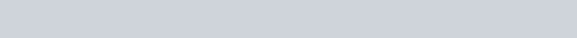# Maximum Product Subarray II

Difficulty Level Medium
Array Dynamic ProgrammingViews 1531

## Problem Statement

In the “Maximum Product Subarray II” problem we have given an array consisting of positive, negative integers, and also zeroes. We need to find the maximum product of the subarray.

## Input Format

The first line containing an integer N.

Second-line containing N space-separated integers.

## Output Format

The only one line containing an integer denoting the maximum product of the subarray.

## Constraints

• 1<=N<=10^5
• -100<=A[i]<=100

## Example

```5
3 -6 -1 0 4```
`18`

Explanation: The subarray [3, -6, -1] is having the maximum product.

## Algorithm for Maximum Product Subarray II

We are given an integer array and we have to find the continuous subarray which has the largest product. Before going to the full solution let us see the simplest version of the problem-

• If the array consists of only positive numbers – The solution would be the product of all elements of the array.
• If the array contains non-negative numbers(positive and zero) – We traverse the array and calculate running_product when we encounter a   zero, then running_product will be zero, So whenever running_product is less than the current number,  we consider a new subarray start from that position. We also need a variable to store the maximum running product that we encounter.

Int mx_product = array

Int running_product = array

for(int i=1;i<array.size;i++){

running_product = max(running_product*array[i],array[i]);

mx_product = max(mx_product,running_product)

}

• Now, consider negative numbers also – Consider an arrayWhen we are at the second element our running_product becomes -12. But at 3rd position, it becomes 60. When we encounter negative numbers the sign of our product changes. The product becomes really small and then becomes large on sign change. So we take two running product variables running_prod_max and running_prod_min. Whenever we encounter a negative number we swap the running_prod_max and running_prod_min because If (a <= b)

If we multiply both by -1

Then  -a>=b

running_prod_max will store the running product and update it when the running product is maximum than running_prod_max similarly, we update the running_prod_min when the running product is less than running_prod_min.

We also take a variable max_prod to store the maximum product.

## Implementation

### C++ Program for Maximum Product Subarray II

```#include <iostream>
using namespace std;

int max_prod_subarray(int a[],int n){
if(n==0) return 0;

int max_prod = a, running_prod_max = a, running_prod_min = a;

for(int i=1;i<n;i++){
if(a[i]<0){
swap(running_prod_max,running_prod_min);
}
running_prod_max = max(running_prod_max*a[i],a[i]);
running_prod_min = min(running_prod_min*a[i],a[i]);

max_prod = max(max_prod,running_prod_max);
}

return max_prod;

}

int main() {
int n;
cin>>n;
int arr[n];
for(int i=0;i<n;i++) cin>>arr[i];
int max_prod = max_prod_subarray(arr,n);
cout<<max_prod<<endl;
return 0;
}```

### Java Program for Maximum Product Subarray II

```import java.util.*;
import java.lang.*;
import java.io.*;

public class Main
{
public static void main (String[] args) throws java.lang.Exception
{
Scanner sc = new Scanner(System.in);
int n = sc.nextInt();
int[] arr = new int[n];
for(int i=0;i<n;i++){
arr[i]=sc.nextInt();
}
int max_prod = max_prod_subarray(arr,n);
System.out.println(max_prod);
}

public static int max_prod_subarray(int[] a,int n){
if(n==0){
return 0;
}
int max_prod = a, running_prod_max = a, running_prod_min = a;

for(int i=1;i<n;i++){
if(a[i]<0){
swap(running_prod_max,running_prod_min);
}
running_prod_max = Math.max(running_prod_max*a[i],a[i]);
running_prod_min = Math.min(running_prod_min*a[i],a[i]);

max_prod = Math.max(max_prod,running_prod_max);
}

return max_prod;
}

public static void swap(int a, int b)
{
int temp = a;
a = b;
b = temp;
}

}```
```4
1 -2 -3 4```
`24`

## Complexity Analysis for Maximum Product Subarray II

### Time Complexity

O(n) where n is the size of the given array. Here we traverse the array only one time and get the answer in only one traversal.

### Space Complexity

O(1) because we don’t create any auxiliary space here.

Translate »